# KS1 maths curriculum: the ultimate guide

Explore the whole KS1 maths curriculum, including the topics covered and when children learn about them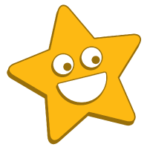Author
Lucy Hart

Last updated: July 3rd, 2023# KS1 maths curriculum: the ultimate guide​

Explore the whole KS1 maths curriculum, including the topics covered and when children learn about them!Author
Lucy Hart

Last updated: July 4, 2023# KS1 maths curriculum: the ultimate guide​

Explore the whole KS1 maths curriculum, including the topics covered and when children learn about them!Author
Lucy Hart

Last updated: July 4, 2023

# KS1 maths curriculum: the ultimate guide​

Explore the whole KS1 maths curriculum, including the topics covered and when children learn about them!Author
Lucy Hart

Last updated: July 4, 2023

Maths is a truly amazing subject. Not only is it invaluable for school, but it can also help learners develop their problem solving and critical thinking skills!

With this in mind, understanding the KS1 maths curriculum is a great way to support your child’s learning journey. So in this guide, we’ll explore the KS1 maths curriculum, including what topics children learn and when. Let’s get started!

## Overview of the KS1 maths curriculum

The KS1 maths curriculum covers a variety of topics that are designed to develop each child’s mathematical knowledge and give them the skills they need for KS2.

As outlined by the national curriculum, these topics are:

• Number: number and place value
• Number: multiplication and division
• Number: fractions
• Measurement
• Geometry: properties of shapes
• Geometry: position and direction
• Statistics

Now, let’s explore each area in a little more detail!

### Number: number and place valueAs numbers form the foundation of mathematical problems, understanding what they are and how to use them is really important.

In the KS1 maths curriculum, children learn to count, read, write and represent numbers up to 100. They also learn how to compare numbers and use the place value system.

Year 1

• Count to and across 100, forwards and backwards, from any given number
• Count, read and write numbers up to 100 in numerals
• Read and write numbers from 1 to 20 in numerals and words
• Count in multiples of twos, fives and tens
• Identify one more and one less than a given number
• Identify and represent numbers using objects and visual representations
• Use the following language: ‘equal to’, ‘more than’, ‘less than’, ‘most’ and ‘least’

Year 2

• Count in steps of 2, 3 and 5 from 0, and in 10s from any number, forward and backwards
• Recognise the place value of each digit in a two-digit number
• Identify, represent and estimate numbers using different representations
• Compare and order numbers from 0 to 100, using <, > and = signs
• Read and write numbers to 100 in numerals and in words
• Use place value and number facts to solve problems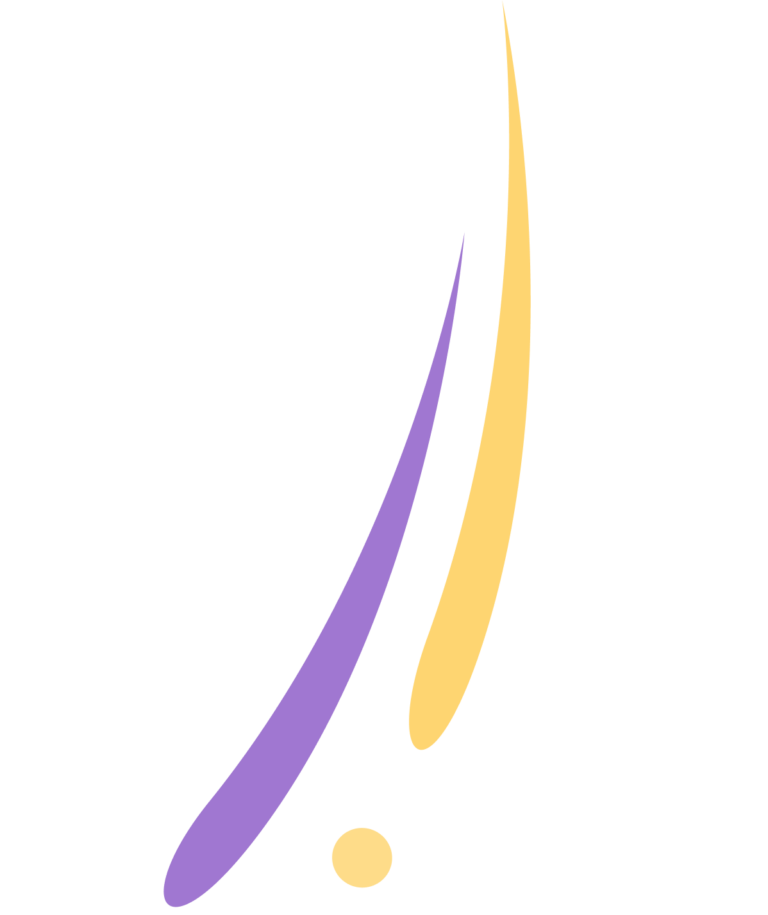Number and place value activities

• Count and spot numbers in everyday situations, like counting steps or toys
• Use items from around the house to help your child visualise numbers and place value, such as LEGO bricks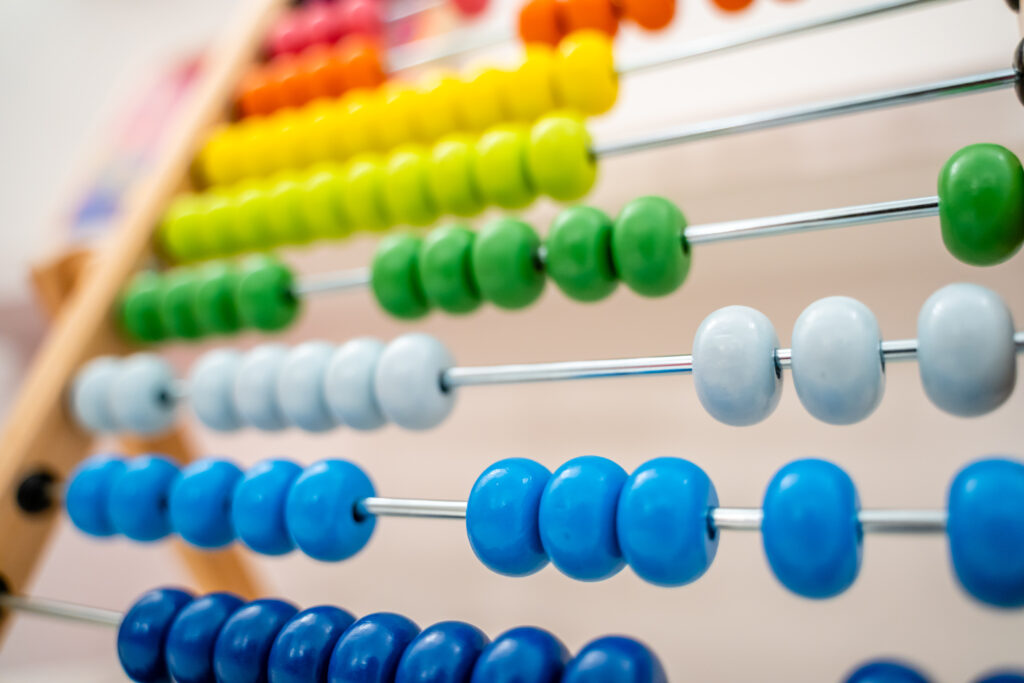Addition and subtraction are key when it comes to problem solving.

In KS1 maths, children learn various strategies for mental and written addition and subtraction. They also explore number bonds, counting techniques and inverse operations.

Year 1

• Read, write and interpret statements involving addition, subtraction and = signs
• Represent and use number bonds and subtraction facts within 20
• Add and subtract one-digit and two-digit numbers to 20, including zero
• Solve one-step and missing number problems that involve addition and subtraction, using objects and pictorial representations

Year 2

Solve problems with addition and subtraction:

• using concrete objects and pictorial representations, including those representing numbers, quantities and measures
• applying their knowledge of mental and written method
• Recall and use addition and subtraction facts to 20, and use related facts up to 100

Add and subtract numbers using concrete objects, pictorial representations and mentally, including:

• a two-digit number and ones
• a two-digit number and tens
• two two-digit numbers

• Show that addition of two numbers can be done in any order and subtraction of one number from another can’t
• Recognise and use the inverse relationship between addition and subtraction, and use this to check calculations and solve missing number problems

Tips to explore addition and subtraction:

• Play card and dice games that involve adding and subtracting numbers
• Bring maths into real-life situations, such as sharing snacks or counting items during a shopping trip

## Explore the KS1 maths curriculum with DoodleMathsLooking for ways to bring the KS1 math curriculum to life? If so, DoodleMaths is here to help!

Created by our team of teachers, DoodleMaths is an award-winning app that’s filled with interactive questions and games that explore all KS1 maths topics.

Designed to be used ‘little and often’, it creates every child a unique learning experience tailored to their needs, boosting their confidence and ability in maths. And best of all, you can try it for free!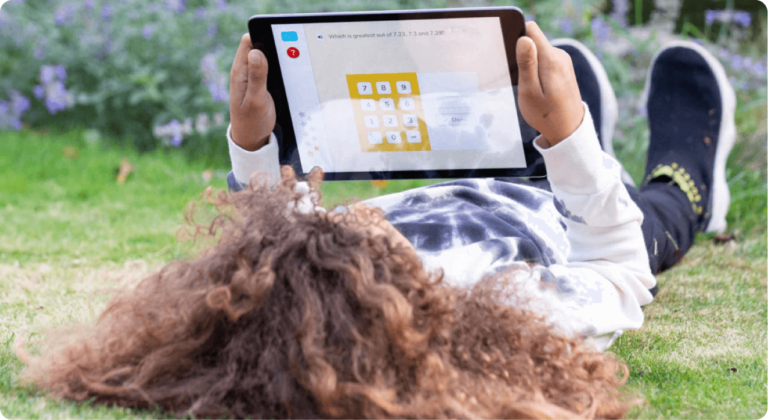### Number: multiplication and division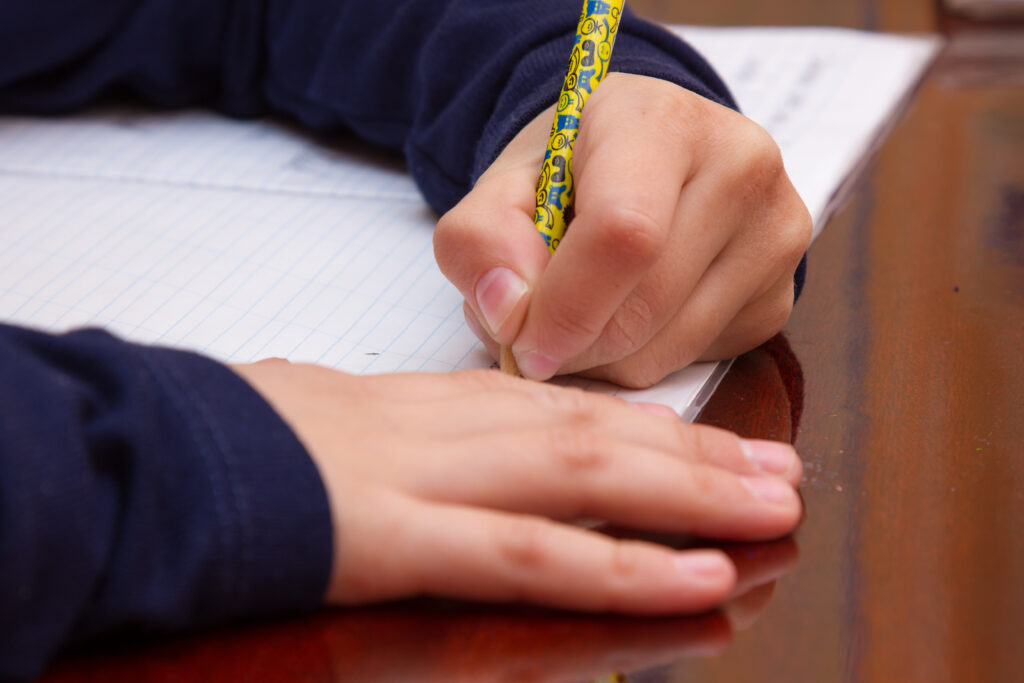In the KS1 maths curriculum, children build on their knowledge of addition and subtraction by exploring multiplication and division.

As part of this, they learn how to solve problems using multiplication and division facts, arrays and pictorial representations.

Year 1

• Solve one-step problems involving multiplication and division by calculating the answer using concrete objects, pictorial representations and arrays

Year 2

• Recall and use multiplication and division facts for the 2, 5 and 10 multiplication tables, including recognising odd and even numbers
• Calculate mathematical statements for multiplication and division within the multiplication tables and write them using the x, ÷ and = signs
• Show that multiplication of two numbers can be done in any order and division of one number by another can’t
• Solve problems involving multiplication and division, using arrays, repeated addition, mental methods, and multiplication and division facts

More on multiplication and division

### Number: fractions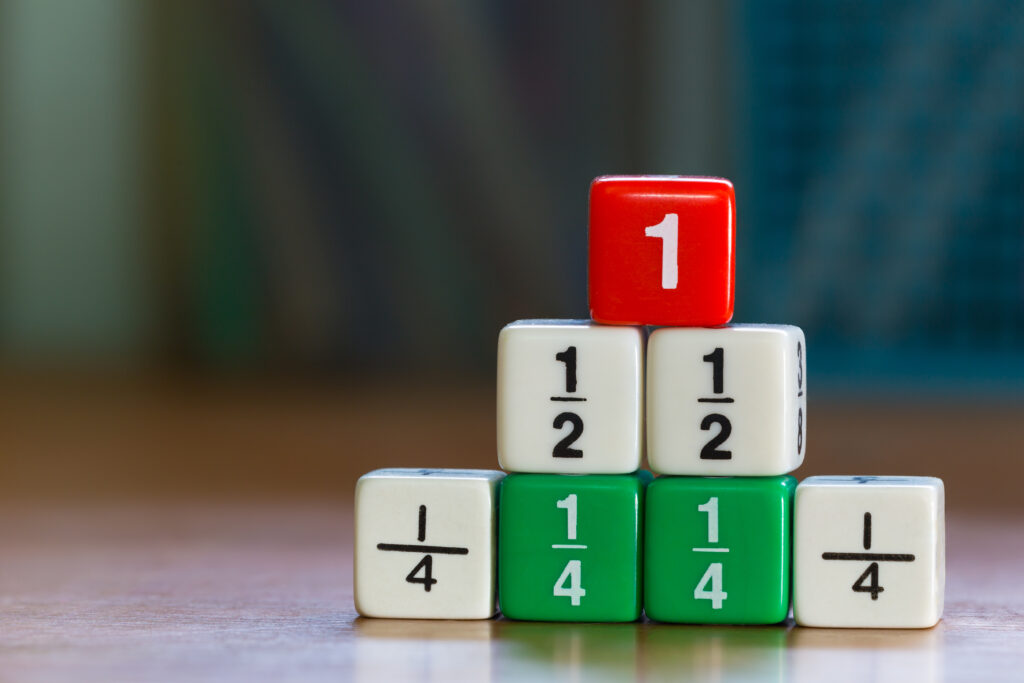Fractions are another important skill that children explore in the KS1 maths curriculum.

Not only can they be used in the classroom, but they’re also very handy for real life!

Year 1

• Recognise, find and name a half as one of two equal parts of an object, shape or quantity
• Recognise, find and name a quarter as one of four equal parts of an object, shape or quantity

Year 2

• Recognise, find, name and write fractions 1/3, 1/4, 1/2 and 3/4 of a length, shape, set of objects or quantity
• Write simple fractions and recognise the equivalence of 2/4 and 1/2

Tips for learning fractions:

• Encourage your child to arrange real-life quantities into different fractions, such as pizza or apple slices### Measurement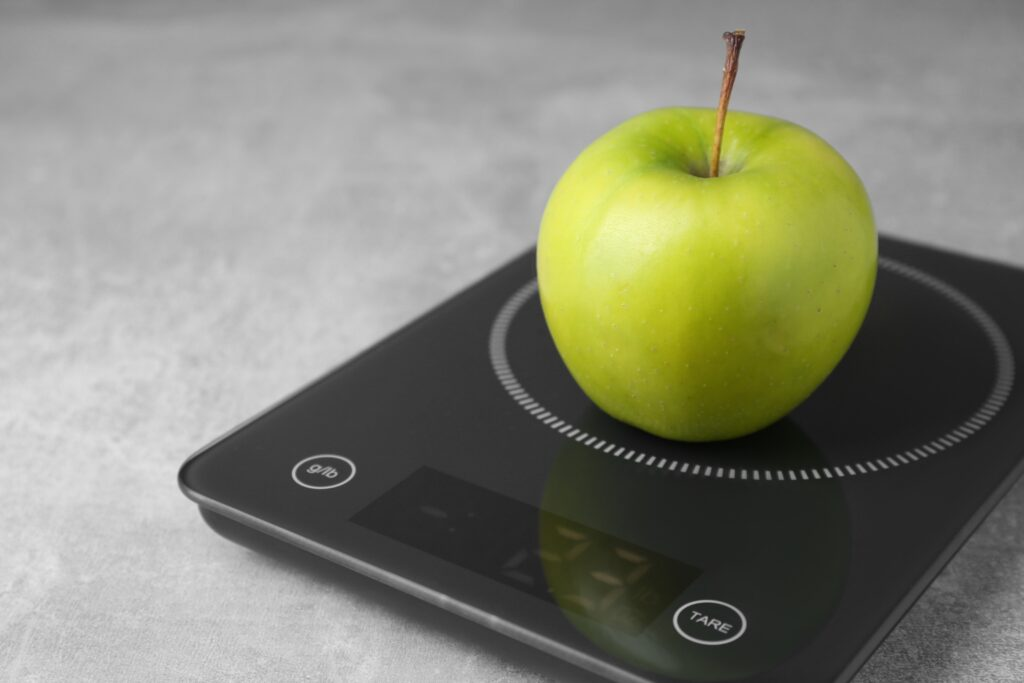Measurement involves comparing a variety of different attributes, such as length, capacity and time.

In the KS1 maths curriculum, children learn how to use appropriate units, estimate measurements and read scales.

Year 1

Compare, describe and solve problems for:

• lengths and heights
• mass/weight
• capacity and volume
• time

Measure and begin to record the following:

• Lengths and heights
• Mass/weight
• Capacity and volume
• Time (hours, minutes, seconds)

• Recognise and know the value of different denominations of coins and notes
• Sequence events in chronological order
• Recognise and use language relating to days of the week, months and years
• Tell the time to the hour and half past the hour, and draw the hands on a clock face to show these times

Year 2

• Use appropriate units to estimate and measure length, mass, temperature and capacity to the nearest unit using rulers, scales, thermometers and measuring vessels
• Compare and order lengths, mass, volume and record the results using >, < and =
• Recognise and use symbols for pounds and pence
• Combine amounts to make a particular value
• Find different combinations of coins that equal the same amounts of money
• Solve simple problems in a practical context involving addition and subtraction of money of the same unit
• Compare and sequence intervals of time
• Write the time to five minutes and draw the hands on a clock face to show these times
• Know the number of minutes in an hour and the number of hours in a day

• Involve your child in cooking or baking activities where they can measure ingredients

### Geometry: properties of shapes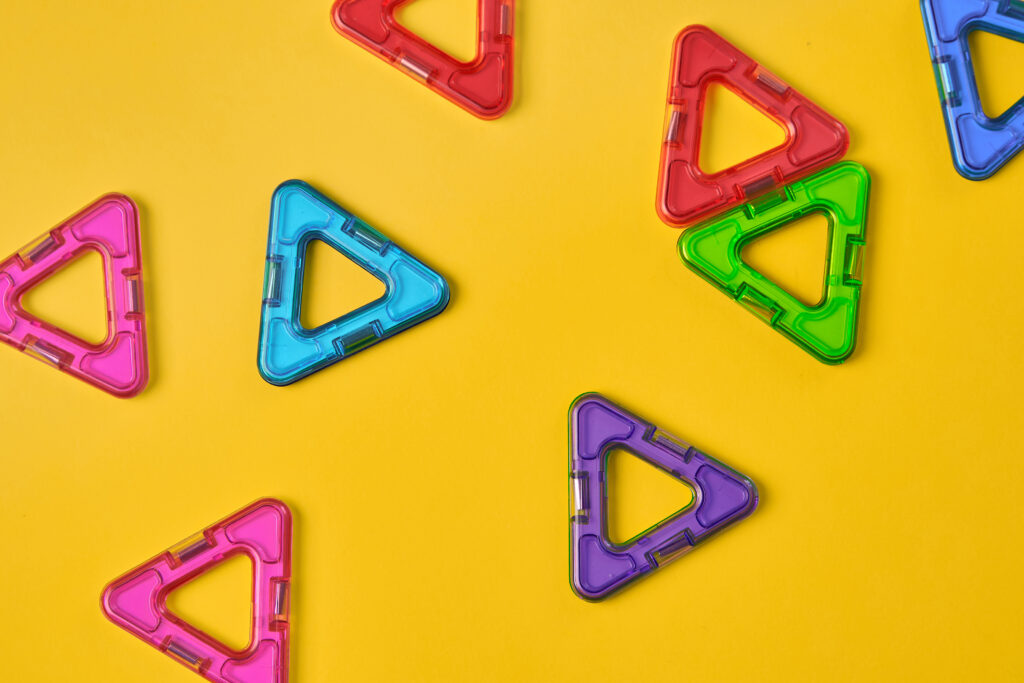Geometry teaches learners all about 2D and 3D shapes, including symmetry, position and direction.

They explore the fundamentals of shapes in the KS1 maths curriculum, including how to recognise and name different types of shapes.

Year 1

Recognise and name common 2D and 3D shapes, including:

• 2D shapes (such as rectangles, circles and triangles)
• 3D shapes (such as cuboids, pyramids and spheres)

Year 2

• Identify and describe the properties of 2D shapes
• Identify and describe the properties of 3D shapes
• Identify 2D shapes on the surface of 3D shapes
• Compare and sort common 2D and 3D shapes and everyday objects

Tips for learning about properties of shapes:

• Use building blocks or Playdough to create and explore different shapes together
• Take walks and hunt for shapes in the environment

### Geometry: position and directionWith a firm knowledge of position and direction, your child will not only be able to navigate the maths curriculum, but it’ll also help them with real-life orientation!

Year 1

• Describe position, direction and movement, including whole, half, quarter and three-quarter turns

Year 2

• Order and arrange combinations of mathematical objects in patterns and sequences
• Use mathematical vocabulary to describe position, direction and movement, including movement in a straight line and distinguishing between rotation as a turn and in terms of right angles for quarter, half and three-quarter turns

Tips for learning about geometry and direction:

• Head outside and challenge your child to turn in different directions, such as making a three-quarter turn

### Statistics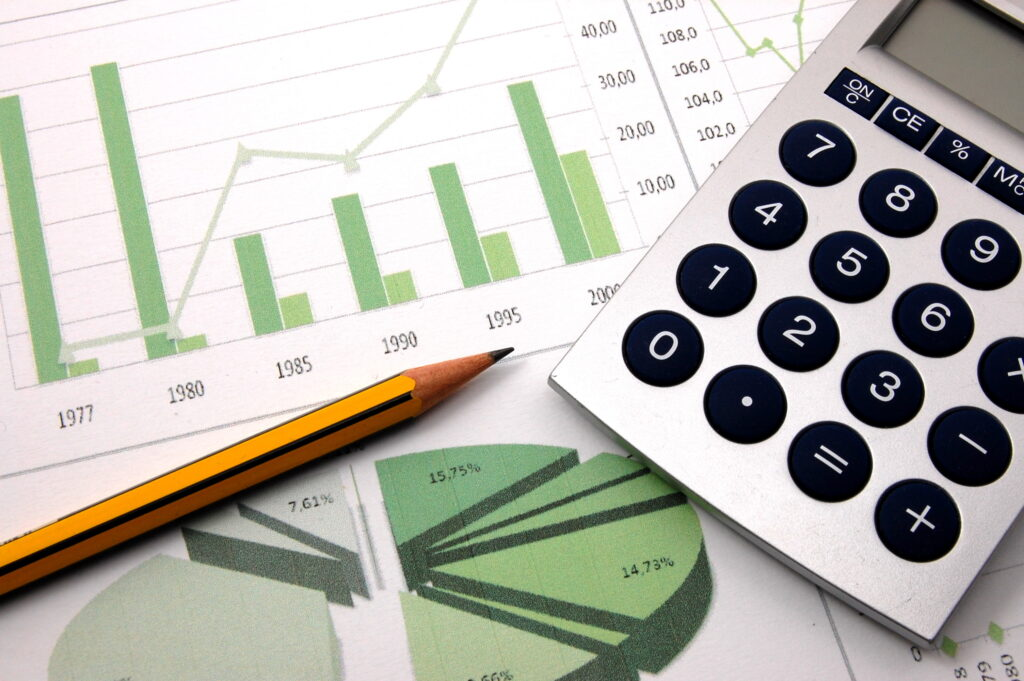Data detectives, assemble! In the KS1 curriculum, children learn how to collect, organise and interpret data.

They also explore how to gather information, create graphs and interpret data using pictograms, tally charts and block graphs.

Year 1

• Interpret and construct simple pictograms, tally charts, block diagrams and tables
• Ask and answer questions by counting the number of objects in a category and sorting the categories by quantity

Year 2

• Interpret and present data using bar charts, pictograms and tables
• Solve one-step and two-step questions using information presented in scaled bar charts and pictograms and tables

• Conduct simple surveys or polls at home or in the classroom and create graphs to represent the data
• Explore everyday situations where data is presented, such as weather forecasts or sports statistics

### In summary...

And there we have it – a complete guide to the KS1 maths curriculum! With this handy summary to refer back to, you’re all set to support your child’s mathematical adventure.

Finally, if you’re looking for ways to bring the curriculum to life, DoodleMaths has you covered.

With interactive activities to enjoy, educational games to play and rewards to unlock, your child will always look forward to maths practice – and you can try it for free!Meet DoodleMaths, the app that's proven to boost ability in maths!# Are you a parent, teacher or student?

Are you a parent or teacher?

## Hi there!

Book a chat with our team